# Arc command

Draws an arc from center, start and end points, tangent, radius, and direction.

Where can I find this command?
Toolbars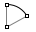Main1 and Arc toolbars
Curve > Arc >
Center, Start, Angle
Start, End, Direction
Start, End, Point
Start, Point, End
Tangent to Curves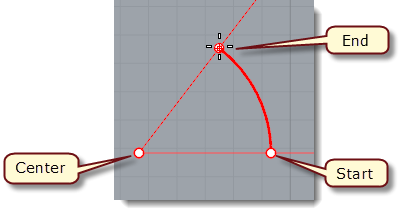#### To draw an arc with the default center, start, end option

1. Pick the center of the arc's radius.
2. Pick the arc's first endpoint. This point is also the arc's start point.
3. Pick the arc's second end point or type an angle.
Arc command options
Deformable
The Deformable option draws a NURBS approximation of an arc that has control points making it deformable by editing its control points. Two settings are added to control the degree and point count of the arc.Deformable settings
Degree
Specifies the degree of the curve (or surface). When drawing a high-degree curve, the output curve will not be the degree you request unless there is at least one more control point than the degree.

PointCount
Specifies the number of control points.

Tilted
The Tilted option draws an arc whose plane is tilted from the current construction plane. The option is available after you pick the Center location for the default arc starting from the center.

Tilted steps
1. Pick the center of the arc.
2. At the Start of arc prompt, click the Tilted option.
3. At the Start of tilted arc prompt, pick a location for the first endpoint of the arc. A plane of reference is drawn for the arc's plane.
4. In a different viewport or using elevator mode, specify the arc's orientation.
StartPoint
Draws an arc starting from the arc's first end point.

StartPoint Steps
1. Pick the arc's start point.
2. Pick the arc's end point.
3. Click a location the arc passes through.
StartPoint options
Direction
Specifies the direction of a line tangent to the arc's first end point.

Direction steps
1. Pick a start point.
2. Pick a tangent direction.
3. Pick an end point.
ThroughPoint
Specifies a location the arc curve must pass through.

ThroughPoint steps
1. Pick a start point.
2. Pick a location.
3. Pick an end point.
Center
Specifies a center point for the arc's radius.

Center steps
• Pick a center point.
Center command options
Length
Sets the arc length, measured along the arc.

Direction
Sets the direction for drawing the arc.

Counterclockwise
Draws the arc in a counterclockwise direction from the startpoint.

Clockwise
Draws the arc in a clockwise direction from the startpoint.

Tangent
Draws an arc tangent to two curves with an option to specify a radius.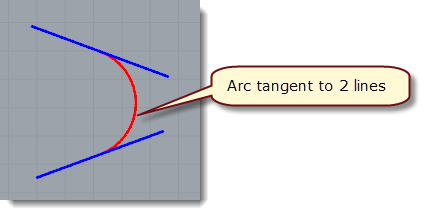Tangent steps
1. Select a tangent curve.
2. Select the second tangent curve, or type a radius to restrict the arc to the specified radius. If a tangent point exists on the second curve that meets the radius requirement, the tangent constraint will appear at that point as you drag the arc around.
3. Select a third tangent curve, or press Enter to draw a circle.
4. Click to choose the arc direction from the options displayed when the mouse is dragged.
Tangent options
Point
Releases the command from the tangent constraint. Pick a point not on the tangent curve for the arc to pass through.

FromFirstPoint
Forces the arc to go through the first picked point on the curve instead of allowing the point to slide along the curve.

Specifies a radius. If a tangent point exists on the second curve that meets the radius requirement, the tangent constraint will appear at that point as you drag the circle.

Extension
Extends any curve with an arc by picking the end location of the arc extension.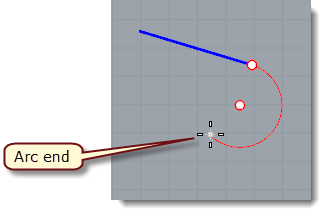Extension steps
1. Pick the curve to extend.
2. Pick the location where you want the arc to end. You can type a positive or negative number or pick two points to specify the distance from the endpoint of the curve to the endpoint of the arc.
Extension option
Center
Extends a curve with an arc by picking the arc's center and endpoint.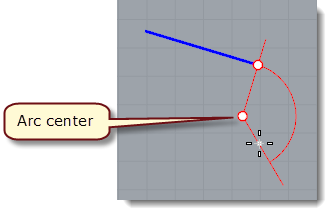Center steps

1. Pick the arc's center point. You can type a positive or negative number or pick two points to specify the distance from the endpoint of the curve to the center of the arc.
2. Pick the arc's end point or angle.
Notes
• The arc extension is not joined to the original curve.
• To draw an arc extension and join it in one step, use the Extend command.
• The center point is constrained to a plane perpendicular to the curve end. If the extension is drawn in a view perpendicular to the constraint plane, the input will be automatically locked parallel to the construction plane.
• In other views Rhino tries to make sure one of the ortho directions is parallel to the construction plane. Press the Shift key when making the pick to maintain the ortho direction.